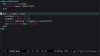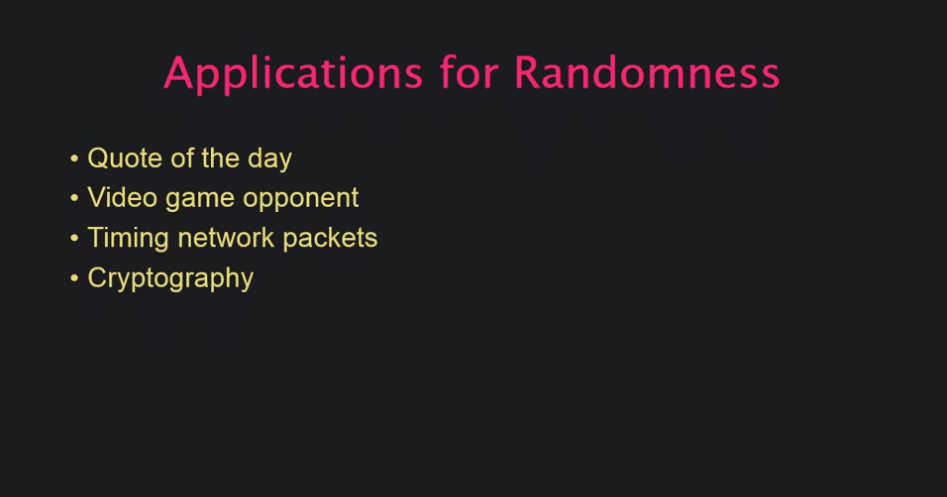In Progress
Unit 1, Lesson 1
In Progress

# RandomnessVideo transcript & code

The other day we talked a bit about picking things at random in Ruby. I thought today we could dig a litter deeper into the notion of randomness.

Making random choices is an important capability in many types of program. Whether it's choosing a "quote of the day", making sure a video game opponent isn't too predictable, or keeping network packets from colliding, we need to be able to effectively "roll the dice" in our programs. Perhaps nowhere is this more true than in cryptographic code, where being able to generate sets of truly random numbers is essential.Unfortunately, computers are not good at generating random numbers. Scratch that; strictly speaking, computers are flat-out incapable of generating truly random values. By definition, a computer is a deterministic machine. The term for a CPU that generates unpredictable values is a "broken CPU".But we need random numbers. In the most exacting of applications, where only the purest chaos will do, computers are connected to dedicated random number generator devices, which derive their data by observing some kind of natural process like atmospheric radio noise or cosmic background radiation.Barring physical device support, operating systems and programming languages fall back on "pseudorandom" number generation in order to generate random numbers. This process has two parts.

First, the machine collects "entropy", that is, it collects some data from various sources that are unlikely to be predictable. For instance, the Linux kernel uses data generated by device driver events. Modern computers are constantly receiving input from a variety of sources, such as incoming network packets, the user moving the mouse, and so on. It is extraordinarily unlikely that someone could reproduce the exact sequence of input events that occurred for a given machine at a given time, so this is a reasonably good source of entropy.Of course, for any given period of time, there are a limited number of unpredictable hardware inputs coming into a given machine. These inputs are known as the machine's "entropy pool", and every time a program requests some random values, that pool is drained a little bit.

In order to create unlimited quantities of pseudorandom data, operating systems and programming languages use this entropy pool as an input into a second phase of generation. The initial, "high quality" random numbers are fed into an algorithm which is known to have good pseudorandom properties. Once the algorithm is seeded with some initial random data, it can generate an unlimited stream of numbers.

Let's take a look at the raw Linux random number source. We can do this by reading some bytes from `/dev/urandom`, then unpacking the results as a series of integers.

``````File.read("/dev/urandom", 8).unpack("C*") # => [15, 32, 166, 42, 14, 92, 188, 3]
``````

So what does it actually mean for an algorithm to have good random properties? It means that for any given random seed, the successive values generated by the algorithm will not have any recognizable or predictable sequence or pattern.

Let's look at an example of what this means. We'll invent our own, absolutely terrible, psuedorandom algorithm. It will take a seed, treat it as a 32-bit integer, and simply do a binary left rotation to the integer every time a new random number is requested. Optionally, the `#rand` method will take a `max` argument, in which case the output will be modulo the max.

If we seed this with the value 0xFFFF, and then successively request random numbers and format them in base 2, we can see the rotation in action.

``````class BadRandom
def initialize(seed)
@last = seed & 0xFFFFFFFF
end

def rand(max = nil)
shifted = @last << 1
highbit = @last >> 31
@last = (shifted | highbit) & 0xFFFFFFFF
max ? (@last % max) : @last
end
end

"%032b" % br.rand               # => "00000000000000011111111111111110"
"%032b" % br.rand               # => "00000000000000111111111111111100"
"%032b" % br.rand               # => "00000000000001111111111111111000"
"%032b" % br.rand               # => "00000000000011111111111111110000"
"%032b" % br.rand               # => "00000000000111111111111111100000"
"%032b" % br.rand               # => "00000000001111111111111111000000"
"%032b" % br.rand               # => "00000000011111111111111110000000"
"%032b" % br.rand               # => "00000000111111111111111100000000"
"%032b" % br.rand               # => "00000001111111111111111000000000"
"%032b" % br.rand               # => "00000011111111111111110000000000"
"%032b" % br.rand               # => "00000111111111111111100000000000"
"%032b" % br.rand               # => "00001111111111111111000000000000"
"%032b" % br.rand               # => "00011111111111111110000000000000"
"%032b" % br.rand               # => "00111111111111111100000000000000"
"%032b" % br.rand               # => "01111111111111111000000000000000"
"%032b" % br.rand               # => "11111111111111110000000000000000"
"%032b" % br.rand               # => "11111111111111100000000000000001"
``````

One way to visualize the randomness of data is to project the data as points on a graph. Let's use our terrible random number generator to generate some x/y coordinates. To try and keep things a little unpredictable, we'll seed the generator with the current time as an integer. Then we'll generate 1000 coordinates, where each one is somewhere between 0 and 100. We'll plug these into Gnuplot to generate a graph. Don't worry too much about the details of this code; the important thing is that when we execute it, we see a graphical plot of our attempt at random data.

``````require "gnuplot"

def initialize(seed)
@last = seed & 0xFFFFFFFF
end

def rand(max = nil)
shifted = @last << 1
highbit = @last >> 31
@last = (shifted | highbit) & 0xFFFFFFFF
max ? (@last % max) : @last
end
end

xs = []
ys = []
1000.times do
xs << br.rand(100)
ys << br.rand(100)
end

Gnuplot.open do |gp|
Gnuplot::Plot.new( gp ) do |plot|

plot.data << Gnuplot::DataSet.new( [xs, ys] ) do |ds|
ds.with = "points"
ds.notitle
end
end
end
``````

The results look surprisingly sparse.In order to see just how bad our random number generator really is, we actually need to introduce a little bit of properly random jitter from a the system random number generator. With the jitter added, we can see that the reason the first plot looked so sparse is that we were just getting a few points repeated over and over. Now that they have been slightly randomized, we can see this repetition as blobs instead of as singular points.

``````require "gnuplot"

def initialize(seed)
@last = seed & 0xFFFFFFFF
end

def rand(max = nil)
shifted = @last << 1
highbit = @last >> 31
@last = (shifted | highbit) & 0xFFFFFFFF
max ? (@last % max) : @last
end
end

xs = []
ys = []
1000.times do
xs << br.rand(100)
ys << br.rand(100)
end

Gnuplot.open do |gp|
Gnuplot::Plot.new( gp ) do |plot|

plot.data << Gnuplot::DataSet.new( [xs.map{|n| n + rand}, ys.map{|n| n+ rand}] ) do |ds|
ds.with = "points"
ds.notitle
end
end
end
``````

Not only are the same points being repeated over and over, but we can also see that the results are clustered in a couple of roughly diagonal stripes.This is the antithesis of the kind of even, unpredictable distribution we want to see from a random algorithm. Let's compare this plot, to the kind of plot we get when we just use system-generated random numbers.

``````require "gnuplot"

xs = []
ys = []
1000.times do
xs << rand(100)
ys << rand(100)
end

Gnuplot.open do |gp|
Gnuplot::Plot.new( gp ) do |plot|

plot.title  "Ruby Random"

plot.data << Gnuplot::DataSet.new( [xs, ys] ) do |ds|
ds.with = "points"
ds.notitle
end
end
end
``````

The difference couldn't be more stark. This time, we see a chaotic distribution all across the graph.So what kind of random number generator are we using when we invoke the `rand` method? Ruby's default random number generator uses an algorithm called a "Mersenne Twister". It seeds it with data from the system's high-quality random number source, such as `/dev/urandom` on linux. However, only the initial seed comes from this source of high-quality entropy. After that, the algorithm proceeds mechanically.

This is good enough for many applications, such as picking dice rolls in a game. However, it is not considered a good enough source of random data to be used in cryptographic applications.

For cryptographically-secure random data, we have to use ruby's `SecureRandom` class. This class interfaces directly to the operating's source of high-quality random values. There is no seeding in this case, since the operating system continually adds new entropy to its internal random number generator, using the hardware inputs we talked about earlier, like network interfaces and user I/O peripherals.

``````require "gnuplot"
require "securerandom"

xs = []
ys = []
1000.times do
xs << SecureRandom.random_number(100)
ys << SecureRandom.random_number(100)
end

Gnuplot.open do |gp|
Gnuplot::Plot.new( gp ) do |plot|

plot.title  "Secure Random"

plot.data << Gnuplot::DataSet.new( [xs, ys] ) do |ds|
ds.with = "points"
ds.notitle
end
end
end
``````

If we plug `SecureRandom` into our plot, we don't see a noticeable difference from the `Kernel#rand`-generated values.We just have to take it on faith that this operating-system-generated random information is sufficiently chaotic for cryptographic use, unlike the standard `rand` method.

Hopefully this episode has given you a better grasp on the fundamentals of random data generation in Ruby. In a future episode, we'll talk about why we might sometimes prefer a seeded pseudorandom number source, rather than using `SecureRandom`. But I think this is enough for now. Happy hacking!